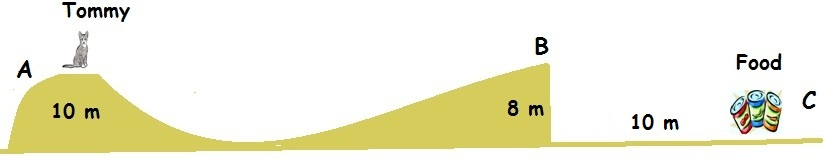Hungry Tommy !!!Tommy, the cat at $A$ is very hungry now and it needs some food which is at $C$. So, it starts sliding from rest at $A$ and reaches $B$ from where it gets projected horizontally towards point $C$. Given that $A$ and $B$ are at heights $\text{10 m, 8 m}$ respectively and the horizontal distance between $B$ and $C$ is $\text{10 m}$. Can Tommy get his food?

Assumptions: All surfaces are friction-less. Tommy gets projected only horizontally at $B$ and not at any angle. Also, he will stop where he lands and will not move further.

Try my World of Physics to solve many problems like this one.

×